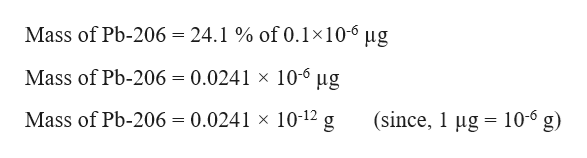Question
31 views

check_circle

Step 1

From the graph, in 2011, the concentration of lead is 0.1 µg/m3 which means 1m3 contains 0.1 µg of lead. Therefore, 1cm3 will contain 0.1×10-6 µg of lead. (since 1 cm3 = 10-6 m3)

Given, lead sample consists of 24.1 % of Pb-206. The mass of Pb-206 in lead sample can be calculated as:help_outlineImage TranscriptioncloseMass of Pb-206 = 24.1% of 0.1x10-6 ug Mass of Pb-206 = 0.0241 x 10-6 ug (since, 1 g 10-6 g) Mass of Pb-206 = 0.0241 x 10-12 g = fullscreen
Step 2

The number of moles of Pb-206 can be calculated as:

Step 3

Since one mole of a component contains 6.022×1023 atoms. Therefore, the number of ...

### Want to see the full answer?

See Solution

#### Want to see this answer and more?

Solutions are written by subject experts who are available 24/7. Questions are typically answered within 1 hour.*

See Solution
*Response times may vary by subject and question.
Tagged in

### General Chemistry